### Home > PC > Chapter 8 > Lesson 8.1.1 > Problem8-12

8-12.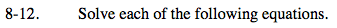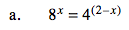Change '8' and '4' to '2' raised to a power. Then set the exponents equal to each other and solve.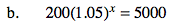Divide both sides by 200 before taking the log of both sides and bringing the 'x' down.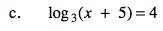Rewrite the equation in exponential form.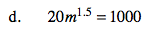$\text{Change '1.5' to }\frac{3}{2}. \text{ Then raise both sides to the power of } \frac{2}{3} \text{ to undo.}$